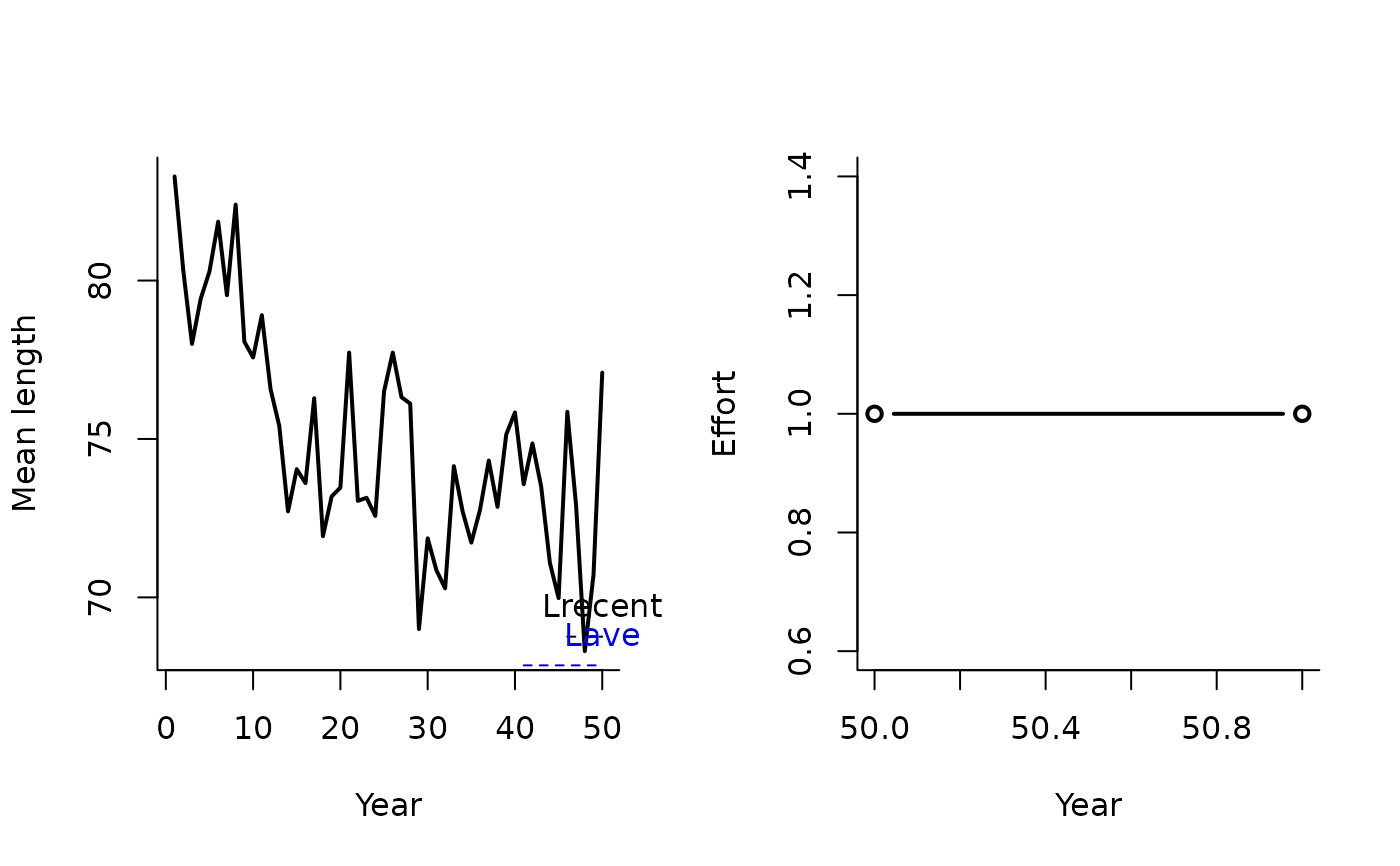A management procedure that incrementally adjusts the total allowable effort (TAE) according to the mean length of recent catches.

LstepCE1(
x,
Data,
reps = 100,
plot = FALSE,
yrsmth = 5,
stepsz = 0.05,
llim = c(0.96, 0.98, 1.05)
)

LstepCE2(
x,
Data,
reps = 100,
plot = FALSE,
yrsmth = 5,
stepsz = 0.1,
llim = c(0.96, 0.98, 1.05)
)

## Arguments

x

A position in the data object

Data

A data object

reps

The number of stochastic samples of the MP recommendation(s)

plot

Logical. Show the plot?

yrsmth

Years over which to calculate trend in mean length.

stepsz

Parameter controlling the size of update increment in effort.

llim

A vector of length reference points that determine the conditions for increasing, maintaining or reducing the effort.

## Value

An object of class Rec-class with the TAE slot(s) populated

## Details

The TAE is calculated as: $$\textrm{TAE} = \left\{\begin{array}{ll} \textrm{TAE}^* - 2 S\textrm{TAE}^* & \textrm{if } r < 0.96 \\ \textrm{TAE}^* - S \textrm{TAE}^* & \textrm{if } r < 0.98 \\ \textrm{TAE}^* & \textrm{if } > 1.058 \\ \end{array}\right.$$ where $$\textrm{TAE}^*$$ is effort in the previous year, $$S$$ is step-size determined by stepsz, and $$r$$ is the ratio of $$L_\textrm{recent}$$ and $$L_\textrm{ave}$$ which are mean length over the most recent yrsmth years and 2 x yrsmth historical years respectively.

The conditions are specified in the llim argument to the function.

## Functions

• LstepCE1: The least biologically precautionary effort-based MP.

• LstepCE2: Step size is increased to 0.1

## Required Data

See Data-class for information on the Data object

LstepCE1: LHYear, ML, MPeff, Year

## Rendered Equations

See Online Documentation for correctly rendered equations

LstepCC1

T. Carruthers

## Examples

LstepCE1(1, Data=MSEtool::SimulatedData, plot=TRUE)
#> Effort
#>      1
LstepCE2(1, Data=MSEtool::SimulatedData, plot=TRUE)#> Effort
#>      1